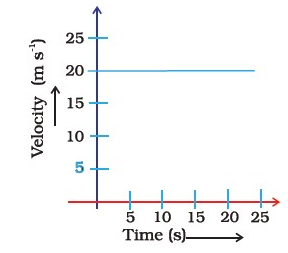#### The velocity time graph (Figure) shows the motion of a cyclist.Find (i) its acceleration (ii) its velocity and (iii) the distance covered by the cyclist in 15 seconds.(i) As there is no change in velocity, we can say that its acceleration is zero all the time.

(ii) If we observe velocity time graph, we can easily deduce that velocity is constant and equal to 20 m/s during complete motion.

(iii) The distance travelled by the cyclist can calculated by the formula:

Hence the distance travelled will be 300 m.